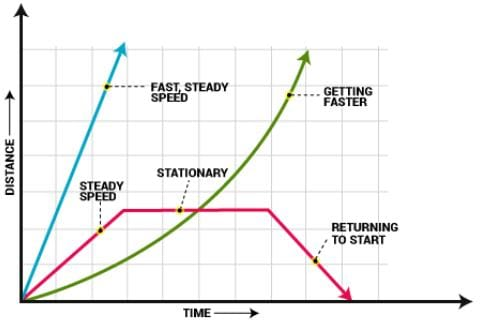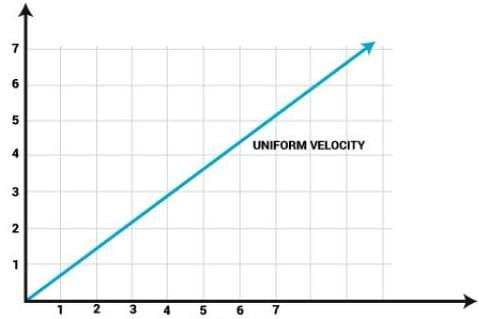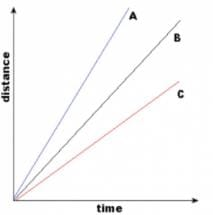Test: Distance-Time Graphs

# Test: Distance-Time Graphs

Test Description

## 5 Questions MCQ Test Science Class 9 | Test: Distance-Time Graphs

Test: Distance-Time Graphs for Class 9 2023 is part of Science Class 9 preparation. The Test: Distance-Time Graphs questions and answers have been prepared according to the Class 9 exam syllabus.The Test: Distance-Time Graphs MCQs are made for Class 9 2023 Exam. Find important definitions, questions, notes, meanings, examples, exercises, MCQs and online tests for Test: Distance-Time Graphs below.
Solutions of Test: Distance-Time Graphs questions in English are available as part of our Science Class 9 for Class 9 & Test: Distance-Time Graphs solutions in Hindi for Science Class 9 course. Download more important topics, notes, lectures and mock test series for Class 9 Exam by signing up for free. Attempt Test: Distance-Time Graphs | 5 questions in 10 minutes | Mock test for Class 9 preparation | Free important questions MCQ to study Science Class 9 for Class 9 Exam | Download free PDF with solutions
 1 Crore+ students have signed up on EduRev. Have you?
Test: Distance-Time Graphs - Question 1

### In a distance-time graph, if the line is horizontal, then the object is:Detailed Solution for Test: Distance-Time Graphs - Question 1

When an object is at rest it will not be able to cover any distance . So for any object at rest the distance -time graph is horizontal to time axis

Test: Distance-Time Graphs - Question 2

### On the distance-time graph, the Y-axis should be labeled as:Detailed Solution for Test: Distance-Time Graphs - Question 2

Independent variables are plotted along X-axis and Dependent variables are plotted along Y-axis. In the distance-time graph, time is independent and distance is a dependent variables.

Test: Distance-Time Graphs - Question 3

### The slope of the distance-time graph is:

Detailed Solution for Test: Distance-Time Graphs - Question 3
The gradient of a distance-time graph represents the speed of an object. The velocity of an object is its speed in a particular direction. The slope on a velocity-time graph represents the acceleration of an object. The distance travelled is equal to the area under a velocity-time graph.

Test: Distance-Time Graphs - Question 4

For a body performing motion with uniform speed, the distance-time graph is:

Detailed Solution for Test: Distance-Time Graphs - Question 4

For a body moving at uniform speed, the distance time graph is a straight line inclined to the time axis, which may pass through origin or some other point on the y- axis.As in uniform motion, the distance time graph would be a straight line, because the equal distance is covered in equal units of time.

Test: Distance-Time Graphs - Question 5

What is the slope of distance-time graph when object is at rest?

Detailed Solution for Test: Distance-Time Graphs - Question 5 Since The Body is at Rest ,It clearly means that The Body hasn't covered any Distance here.So,Distance is equal to zero.So,There is no meaning of having any slope here.. So,The Slope=0 Becoz The Distance at anytime will represent same point in Distance-Time Graph at 0..So,(B)is d correct answer. .

## Science Class 9

115 videos|302 docs|163 tests
 Use Code STAYHOME200 and get INR 200 additional OFF Use Coupon Code
Information about Test: Distance-Time Graphs Page
In this test you can find the Exam questions for Test: Distance-Time Graphs solved & explained in the simplest way possible. Besides giving Questions and answers for Test: Distance-Time Graphs, EduRev gives you an ample number of Online tests for practice

## Science Class 9

115 videos|302 docs|163 tests